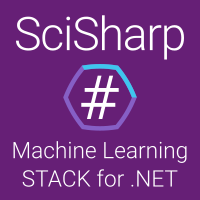NumSharp (NS) is a NumPy port to C# targetting .NET Standard.NumSharp is the fundamental package needed for scientific computing with C# and F#.

Is it difficult to translate python machine learning code into .NET? Because too many functions can’t be found in the corresponding code in the .NET SDK. NumSharp is the C# version of NumPy, which is as consistent as possible with the NumPy programming interface, including function names and parameter locations. By introducing the NumSharp tool library, you can easily convert from python code to C# or F# code. Here is a comparison code between NumSharp and NumPy (left is python, right is C#):### Bold Features

• Use of Unmanaged Memory and fast unsafe algorithms.
• Broadcasting n-d shapes against each other. (intro)
• NDArray Slicing and nested/recusive slicing (`nd["-1, ::2"]["1::3, :, 0"]`)
• Axis iteration and support in all of our implemented functions.
• Full and precise (to numpy) automatic type resolving and conversion (upcasting, downcasting and other cases)
• Non-copy - most cases, similarly to numpy, does not perform copying but returns a view instead.
• Almost non-effort copy-pasting numpy code from python to C#.
• Wide support for `System.Drawing.Bitmap`. (read more)

### Implemented APIs

The NumPy class is a high-level abstraction of NDArray that allows NumSharp to be used in the same way as Python's NumPy, minimizing API differences caused by programming language features, allowing .NET developers to maximize Utilize a wide range of NumPy code resources to seamlessly translate python code into .NET code.

### Install NumSharp in NuGet

``````PM> Install-Package NumSharp
``````

### How to use

``````using NumSharp;

var nd = np.full(5, 12); //[5, 5, 5 .. 5]
nd = np.zeros(12); //[0, 0, 0 .. 0]
nd = np.arange(12); //[0, 1, 2 .. 11]

// create a matrix
nd = np.zeros((3, 4)); //[0, 0, 0 .. 0]
nd = np.arange(12).reshape(3, 4);

// access data by index
var data = nd[1, 1];

// create a tensor
nd = np.arange(12);

// reshaping
data = nd.reshape(2, -1); //returning ndarray shaped (2, 6)

Shape shape = (2, 3, 2);
data = nd.reshape(shape); //Tuple implicitly casted to Shape
//or:
nd =   nd.reshape(2, 3, 2);

// slicing tensor
data = nd[":, 0, :"]; //returning ndarray shaped (2, 1, 2)
data = nd[Slice.All, 0, Slice.All]; //equivalent to the line above.

// nd is currently shaped (2, 3, 2)
// get the 2nd vector in the 1st dimension
data = nd; //returning ndarray shaped (3, 2)

// get the 3rd vector in the (axis 1, axis 2) dimension
data = nd[1, 2]; //returning ndarray shaped (2, )

// get flat representation of nd
data = nd.flat; //or nd.flatten() for a copy

// interate ndarray
foreach (object val in nd)
{
// val can be either boxed value-type or a NDArray.
}

var iter = nd.AsIterator<int>(); //a different T can be used to automatically perform cast behind the scenes.
while (iter.HasNext())
{
int val = iter.MoveNext();

//write
iter.MoveNextReference() = 123; //set value to the next val
//note that setting is not supported when calling AsIterator<T>() where T is not the dtype of the ndarray.
}
``````

### How to run benchmark

``````C: \> dotnet NumSharp.Benchmark.dll nparange
``````

### NumSharp is referenced by

You might also be interested in NumSharp's sister project Numpy.NET which provides a more whole implementation of numpy by using pythonnet and behind-the-scenes deployment of python (read more).

NumSharp is a member project of SciSharp.org which is the .NET based ecosystem of open-source software for mathematics, science, and engineering.

### Regen Templating

Our library contains over 150,000 lines of repetitive generated code, mostly for handling different data types without hurting performance.
The templates can be recognized with `#if _REGEN` blocks and are powered by Regen Templating Engine.
Regen is a powerful external tool (Visual studio extension, download here) that generates on demand based on a C#-like `regen-lang`.

Alternatives To Numsharp
Select To Compare

Alternative Project Comparisons
Related Awesome Lists

Get A Weekly Email With Trending Projects For These Topics
No Spam. Unsubscribe easily at any time.
Python (840,926
C Sharp (170,124
Machine Learning (38,754
Code (18,164
Pandas (6,865
Numpy (6,057
Fsharp (4,162
Tensor (2,375
Ndarray (564
Numsharp (3